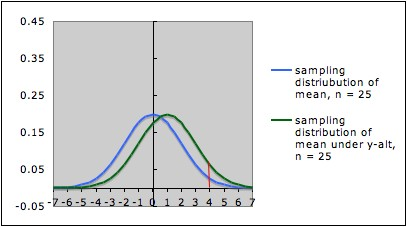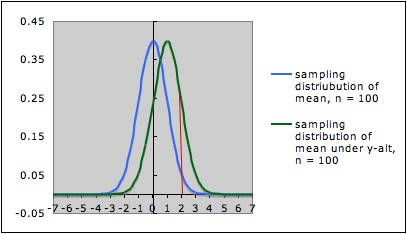COMMON MISTEAKS MISTAKES IN USING STATISTICS: Spotting and Avoiding Them

## Power and Sample Size

Power will depend on sample size as well as on the difference to be detected.

Example: The pictures below each show the sampling distribution for the mean under the null hypothesis µ = 0 (blue -- on the left in each picture) together with the sampling distribution under the alternate hypothesis
µ = 1 (green -- on the right in each picture), but for different sample sizes.
• The first picture is for sample size  n = 25; the second picture is for sample size n = 100.
• Note that both graphs are in the same scale. In both pictures, the blue curve is centered at 0 (corresponding to the the null hypothesis) and the green curve is centered at 1 (corresponding to the alternate hypothesis).
• In each picture, the red line is the cut-off for rejection with alpha = 0.05 (for a one-tailed test) -- that is, in each picture, the area under the blue curve to the right of the red line is 0.05.
• In each picture, the area under the green curve to the right of the red line is the power of the test against the alternate depicted. Note that this area is larger in the second picture (the one with larger sample size) than in the first picture.This illustrates the general situation: Larger sample size gives larger power. The reason is essentially the same as in the example: Larger sample size gives a narrower sampling distribution, which means there is less overlap in the two sampling distributions (for null and alternate hypotheses).

• See Claremont University's Wise Project's Statistical Power Applet for an interactive demonstration of the interplay between sample size and power for a one-sample z-test.
• Sample size needed typically increases at an increasing rate as power increases. (e.g., in the above example, increasing the sample size by a factor of 4 increases the power by a factor of about 2; the graphics aren't accurate enough to show this well.)

### Choosing sample size

The dependence of power on sample size allows us (in principle) to figure out before doing a study what sample size is needed to detect a specified difference, with a specified power, at a given significance level, if that difference is really there. In practice, details on figuring out sample size will vary from procedure to procedure. Some considerations involved:
• The difference used in calculating sample size  (e.g., the specific alternative used in calculating sample size, or the size of confidence interval desired) should be decided on the basis of practical significance and/or "worst case scenario," depending on the consequences of decisions.
• Even when the goal is a hypothesis test, it may be wise to base the sample size on the width of a confidence interval rather than just ability to detect the desired difference: Even when power is large enough to detect a difference, the uncertainty, as displayed by the confidence interval, may still be too large to make the conclusions very credible to a knowledgeable reader.
• Determining  sample size to give desired power and significance level will usually require some estimate of parameters such as variance, so will only be as good as these estimates. These estimates usually need to be based on previous research, experience of experts in the field being studied, and possibly a pilot study. In some cases, it may be wise to use a conservative estimate of variance (e.g., the upper bound of a confidence interval from a pilot study), or to do a sensitivity analysis to see how the sample size estimate depends on the parameter estimate. See Lenth, Russell V. (2001) Some Practical Guidelines for Effective Sample Size Determination, American Statistician, 55(3), 187 - 193 for a discussion of many considerations in deciding on sample size. (An early version and some related papers can be downloaded from his website.)
• Even when there is a good formula for power in terms of sample size, "inverting" the formula to get sample size from power is often not straightforward; it may require some clever approximation procedures. Such procedures have been encoded into computer routines for many common tests. Increasingly, simulations are being used to estimate power and needed sample size.
• Various statistical software packages have power calculations available; however, be sure that the software calculates a priori power, not retrospective power. (See item 7 under Common Mistakes Involving Power.)
• Russell Lenth has online applets for power and sample size for many common experimental designs. His web page also has some suggestions to take into account in calculating sample size.
• See John C. Pezzullo's Interactive Statistics Pages for links to a number of online power and sample size calculators.
• Good and Hardin (2006, Common Errors in Statistics, Wiley, p. 34) report that using the default settings for power and sample size calculations is a common mistake made by researchers.
• For discrete distributions, the "power function" (giving power as a function of sample size) is often saw toothed in shape. A consequence is that software may not necessarily give the optimal sample size for the conditions specified. Good software for such power calculations will also output a graph of the power function, allowing the researcher to consider other sample sizes that might give be better than the default given by the software.

Last updated May 12, 2011